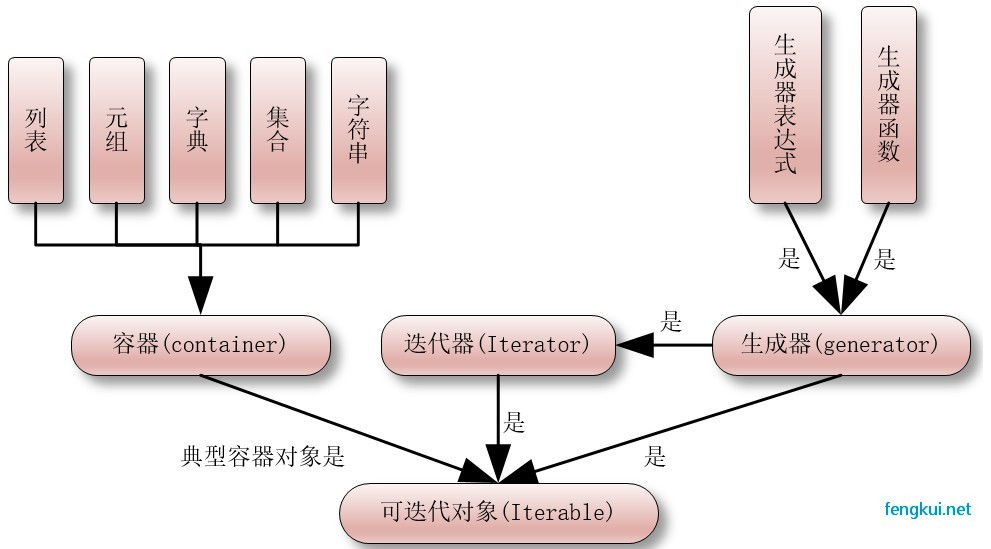# Python3 迭代器与生成器

### 迭代器

``````>>> list=[1,2,3,4]
>>> it = iter(list)    # 创建迭代器对象
>>> print (next(it))   # 输出迭代器的下一个元素
1
>>> print (next(it))
2
``````

``````list=[1,2,3,4]
it = iter(list)    # 创建迭代器对象
for x in it:
print (x)
``````

``````1 2 3 4
``````

``````import sys         # 引入 sys 模块

list=[1,2,3,4]
it = iter(list)    # 创建迭代器对象

while True:
try:
print (next(it))
except StopIteration:
sys.exit()
``````

``````1 2 3 4
``````

### 创建一个迭代器

`__iter__()` 方法返回一个特殊的迭代器对象， 这个迭代器对象实现了 `__next__()` 方法并通过 `StopIteration` 异常标识迭代的完成。
`__next__()` 方法（Python 2 里是 next()）会返回下一个迭代器对象。

``````class MyNumbers:
def __iter__(self):
self.a = 1
return self

def __next__(self):
x = self.a
self.a += 1
return x

myclass = MyNumbers()
myiter = iter(myclass)

print(next(myiter))
print(next(myiter))
print(next(myiter))
print(next(myiter))
print(next(myiter))
``````

``````1 2 3 4 5
``````

### StopIteration

StopIteration 异常用于标识迭代的完成，防止出现无限循环的情况，在 `__next__()` 方法中我们可以设置在完成指定循环次数后触发 StopIteration 异常来结束迭代。

``````class MyNumbers:
def __iter__(self):
self.a = 1
return self

def __next__(self):
if self.a <= 20:
x = self.a
self.a += 1
return x
else:
raise StopIteration

myclass = MyNumbers()
myiter = iter(myclass)

for x in myiter:
print(x)
``````

``````1 2 3 4 5 6 7 8 9 10 11 12 13 14 15 16 17 18 19 20
``````

### 生成器

``````#!/usr/bin/python3

import sys

def fibonacci(n): # 生成器函数 - 斐波那契
a, b, counter = 0, 1, 0
while True:
if (counter > n):
return
yield a
a, b = b, a + b
counter += 1
f = fibonacci(10) # f 是一个迭代器，由生成器返回生成

while True:
try:
print (next(f), end=" ")
except StopIteration:
sys.exit()
``````

``````0 1 1 2 3 5 8 13 21 34 55
``````